Question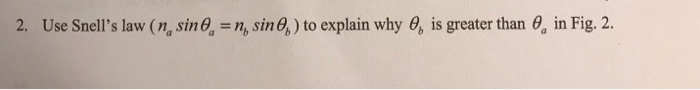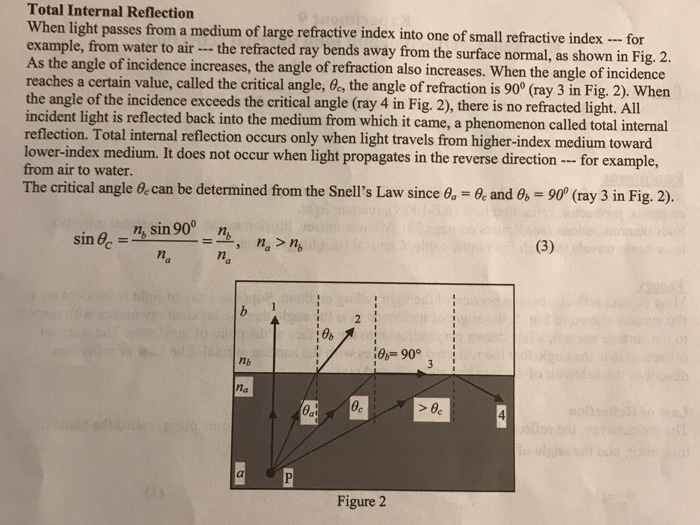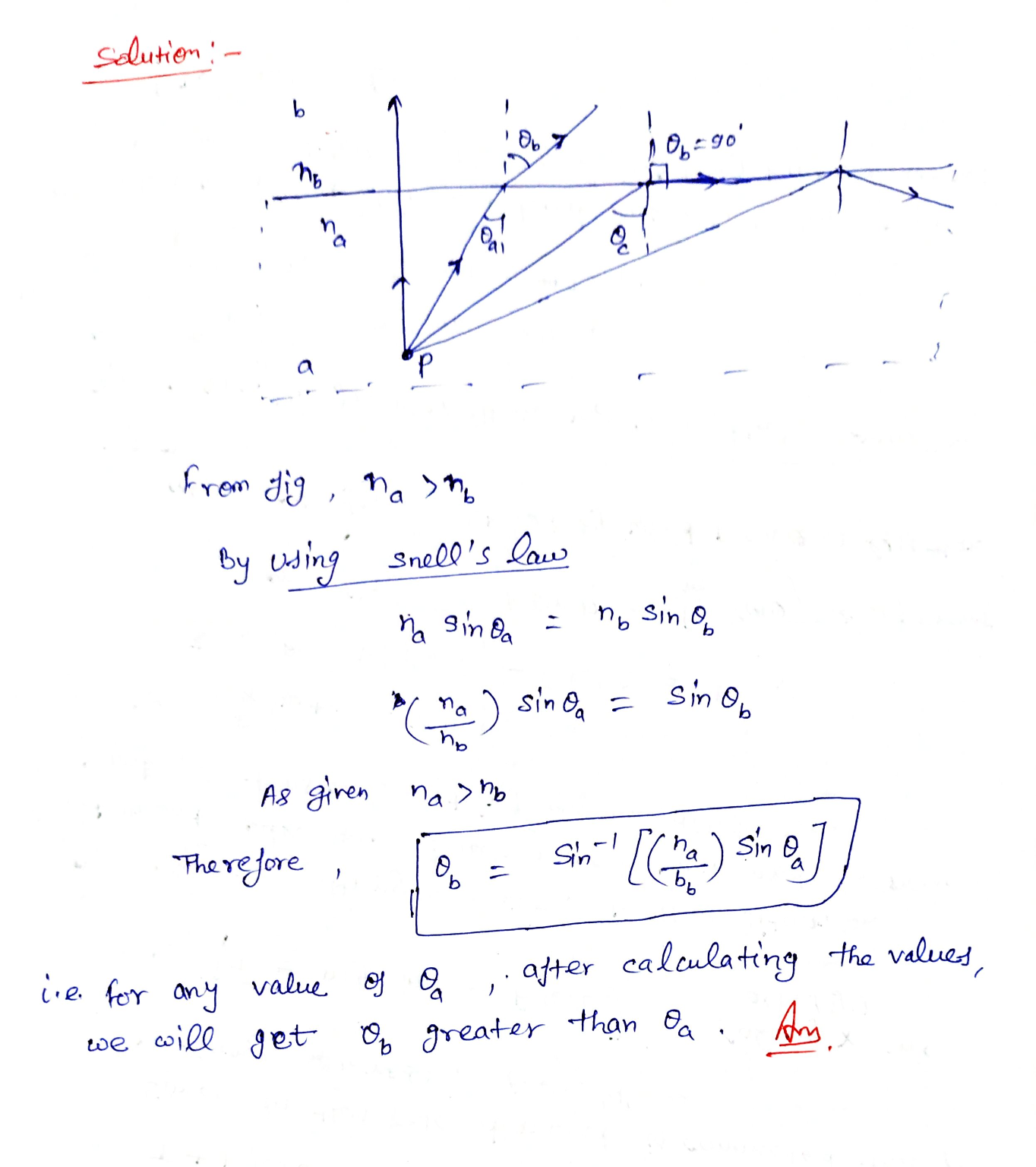Hi any doubt leave a comment... THANKS

#### Earn Coins

Coins can be redeemed for fabulous gifts.

Similar Homework Help Questions
• ### 3. In Fig. 2, if we increase the index n, but keepn, <n,, should the value...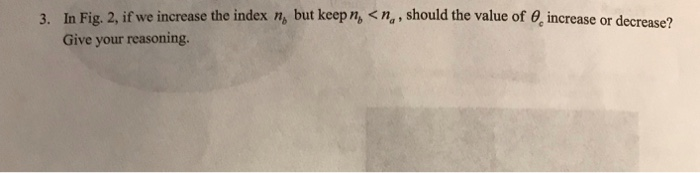3. In Fig. 2, if we increase the index n, but keepn, <n,, should the value of increase or decrease? Give your reasoning. Total Internal Reflection When light passes from a medium of large refractive index into one of small refractive index --- for example, from water to air --- the refracted ray bends away from the surface normal, as shown in Fig. 2. As the angle of incidence increases, the angle of refraction also increases. When the angle of...

• ### Snell's law can be used to solve for the index of refraction of a medium if...

Snell's law can be used to solve for the index of refraction of a medium if the _____ is known. angle of incidence angle of refraction index of refraction of the other medium All of the above . Total internal reflection occurs when the angle of incidence is _____ the critical angle. greater than equal to greater than or equal to None of the above When the slope of a graph is the index of refraction for a material and...

• ### Partner: Date Name 11 Snell's Law Introduction When light passes from one material to another it ...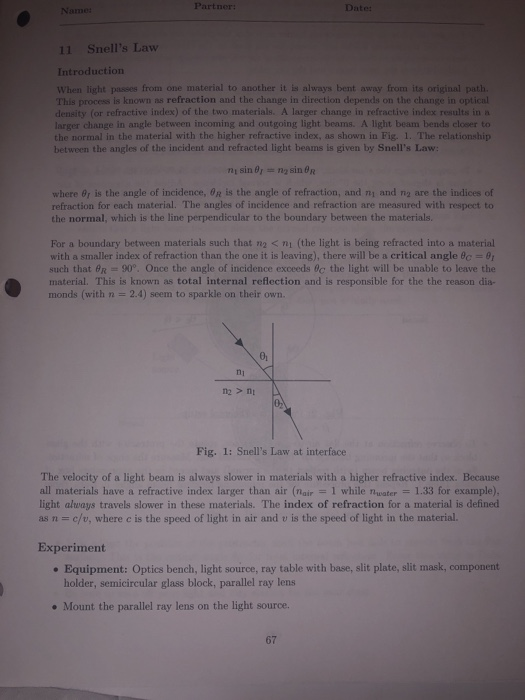Partner: Date Name 11 Snell's Law Introduction When light passes from one material to another it is always bent away from its original path. This process is known as refraction and the change in direction depends on the change in optical density (or refractive index) of the two materials. A larger change in refractive index results in a larger change in angle between incoming and outgoing light beams. A light beam bends closer to the normal in the material with...

• ### 1) Use Snell's Law (na sin(theta)a = nb sin(theta)b) to explain why (theta)b is greater than...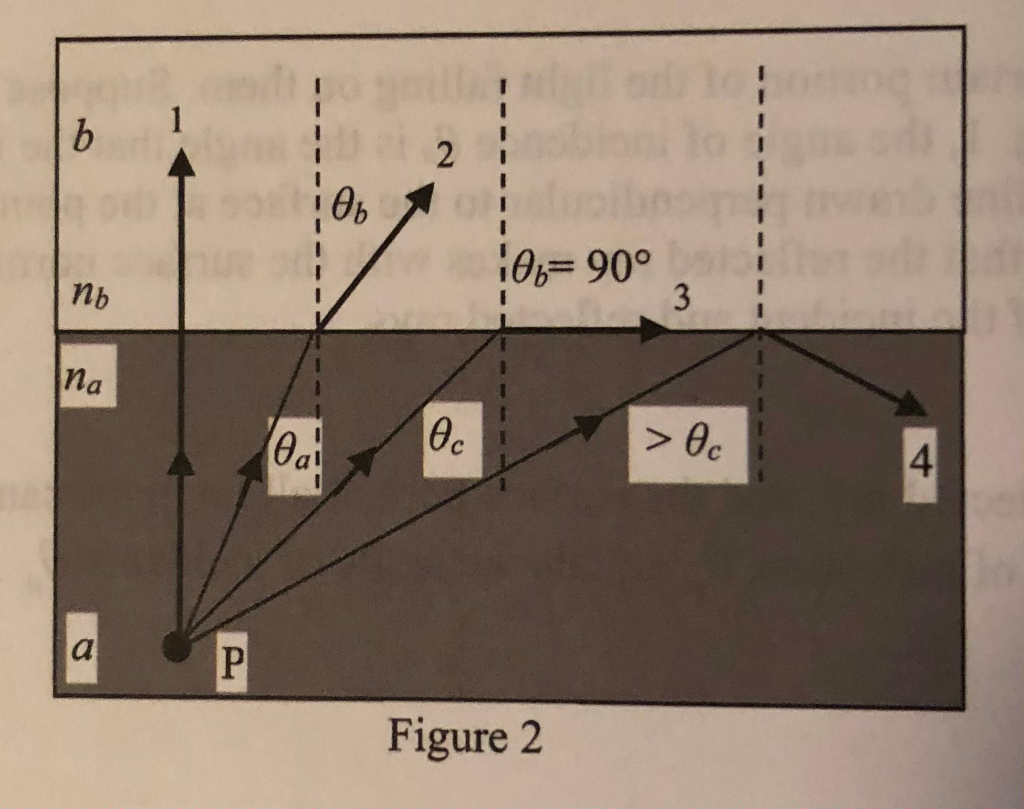1) Use Snell's Law (na sin(theta)a = nb sin(theta)b) to explain why (theta)b is greater than (theta)a in Fig.2 2) In Fig. 2, if we increase the index nb but keep nb < na, should the value of (theta)c increase or decrease? Give your reasoning. 3) Does total internal reflection occur if the refraction of light is passing from ait into water? Why? 1 b 2 Өь Нө = 90° пь 3 na Өa ec > Ө 4 a Р...

• ### Exercise 1: In the refraction of light, Snell's law relates the angle of incidence θǐne to...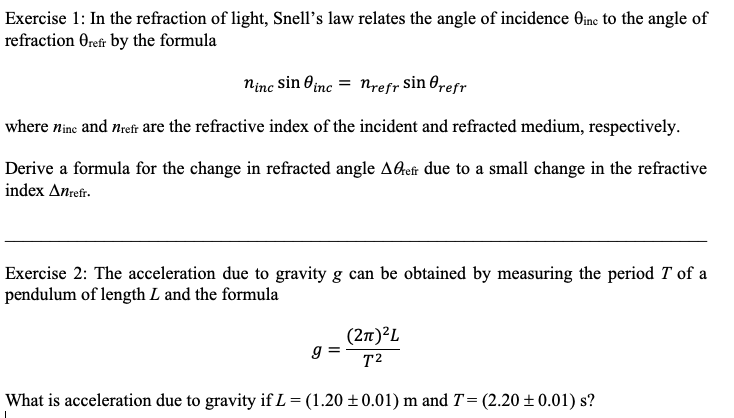Exercise 1: In the refraction of light, Snell's law relates the angle of incidence θǐne to the angle of refraction Orefr by the formula ninc sin θ¡nc-nrefr sin θrefr where nin and nidi are the refractive index of the incident and refracted medium, respectively. Derive a formula for the change in refracted angle AGhefr due to a small change in the refractive index Δnrefr. Exercise 2: The acceleration due to gravity g can be obtained by measuring the period T...

• ### When a light ray travels from a more dense medium to a less dense medium and...

When a light ray travels from a more dense medium to a less dense medium and the light ray intercepts the interface at some angle, the light ray will be deviated away from the normal to the interface.  When the angle of the incident light ray is equal or greater than some critical angle , the light ray will be bent away from the normal unit the angle of refraction becomes 90, and total internal reflection occurs.  The critical angle,  , for two...

• ### 14) A glass prism with an index of refraction of 1.53 sits on a horizontal surface,...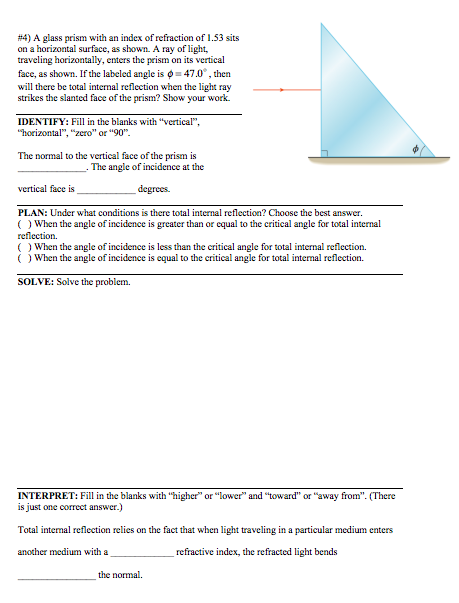14) A glass prism with an index of refraction of 1.53 sits on a horizontal surface, as shown. A ray of light traveling horizontally, enters the prism on its vertical face, as shown. If the labeled angle is O= 470, then will there be total internal reflection when the light ray strikes the slanted face of the prism? Show your work IDENTIFY: Fill in the blanks with "vertical", "horizontal","zero" or "90". The normal to the vertical face of the prism...

• ### Lab Question: Reflection & Refraction (Snell's Law) experiment 1) If you conducted this experiment with a...

Lab Question: Reflection & Refraction (Snell's Law) experiment 1) If you conducted this experiment with a different lens, one with larger refractive index, would you find larger angles of refraction for each angle incidence? Why or why not?? 2) Without refrence to equations, explain why it's physically impossible for total internal reflection (theta= 90 degree) to occur in this experiment ????

• ### 3. The following figure shows light travelling from medium 1 to medium 2. Please draw the...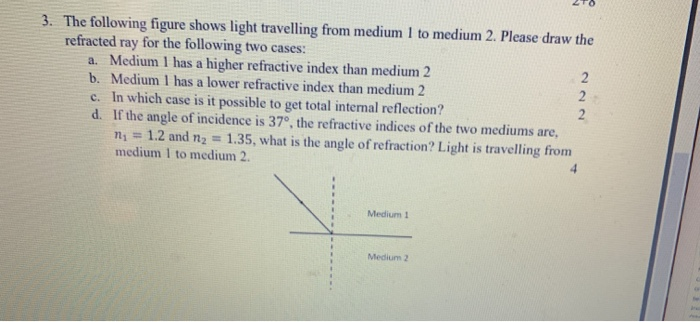3. The following figure shows light travelling from medium 1 to medium 2. Please draw the refracted ray for the following two cases: a. Medium 1 has a higher refractive index than medium 2 b. Medium 1 has a lower refractive index than medium 2 c. In which case is it possible to get total internal reflection? d. If the angle of incidence is 37°, the refractive indices of the two mediums are, n = 1.2 and n2 = 1.35,...

• ### LAB 8: GEOMETRICAL OPTUS: RAY TRACING PART 1 REFRACTION NORMAL FOR THE OPPOSITE PLANE REFRACTED RAY...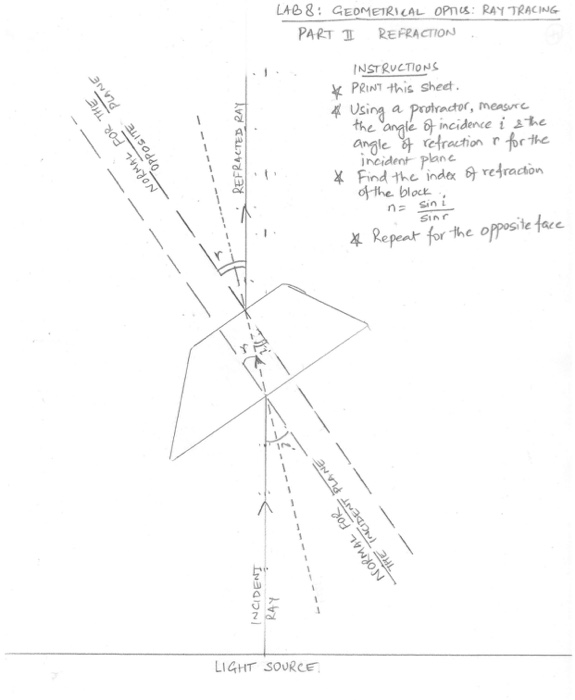LAB 8: GEOMETRICAL OPTUS: RAY TRACING PART 1 REFRACTION NORMAL FOR THE OPPOSITE PLANE REFRACTED RAY INSTRUCTIONS * PRINT this sheet. * Using a protractor, measure the angle of incidence is the angle of refraction & for the incident plane * Find the index of refraction of the block na sini Sin * Repeat for the opposite face A NORMAL FOR THE TROCIDENT PLANE INCIDENT RAY LIGHT SOURCE LAG 8: GEOMETRICAL OPIS: RAY TRONG PART I: CONVEX LENSE INSTRUCTIONS Print...